# Big Picture Machine Learning: Classifying Text with Neural Networks and TensorFlow

TensorFlow is one of the most popular open source AI libraries. Its high in computing efficiency, and the rich development resources make it widely adopted by companies and individual developers.

In this article, the author discusses the main six topics about creating a machine learning model to classify texts into categories:

### 6. How to run the model and get the prediction results

The author also provided the code that can be run in Jupyter notebook. I’ll review these six topics and combine them with my own experience.

## 1. Overview of TensorFlow:

TensorFlow is one of the most popular open source AI libraries. Its high in computing efficiency, and the rich development resources make it widely adopted by companies and individual developers. In my mind, the best way to learn TensorFlow is by using its official website: https://www.tensorflow.org/. In this website, you can go through the “getting started” tutorial and the list for all symbols in TensorFlow.

I will first give you the fundamental definition and the main characteristics of TensorFlow. Tensor is a kind of data structure that can shape the primitive values into an array of any number of dimensions. The rank of tensor is its dimension number. Here, I recommend reading Python’s API for TensorFlow, because it is very friendly for TensorFlow beginner. You should install TensorFlow and configure the environment, just follow the instructions from the official websites. The way to test whether you’ve correctly installed TensorFlow is by importing the library of TensorFlow. In TensorFlow, the computational graph is a core component. The dataflow graph is used to represent the computation process. Under the graph, the Operation is for the units of computation and the Tensor represents units of data. To run the code, we should initialize the Session function. Here is the complete code to execute sum operations:You can see that writing in TensorFlow follows a pattern, and it is easy to remember. You will import the library, create constant tensors, and build the graph. Then we should define which graph will be used in the Session, and define the operation unit. Finally you can use the run() method in Session and evaluate every Tensor, which is passed in the argument fetches.

## 2. The Predictive Model:

Predictive model can be very simple. It combines machine learning algorithm and the data-sets. The process of constructing a model is shown in the figure below:We should first find the correct data as the input, and use some data processing function to manipulate the data. This data is then used to build the model by combining with machine learning algorithms. After you get the model, you can see the model as a predictor and input the data needed to predict, which will yield the result. The process is depicted as the following figure: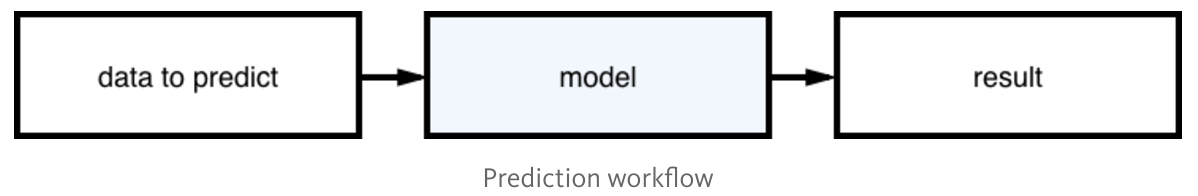For this article, the input is text and the result is the category. This type of machine learning method is called supervised learning, where the training dataset has the texts labeled to which category it belongs. It’s also a classification task and Neural Networks are used to create the model.

## 3. Neural Networks:

The main characteristic of Neural Networks is self-learning, rather than being explicitly programmed. It is inspired by human’s central nervous system. The first neural network algorithm is Perceptron.

To understand the mechanism of how neural network works, the author built a neural network architecture with TensorFlow.

### Architecture of Neural Network:

Here, the author uses 2 hidden layers, and the job of each hidden layers is to transform the inputs into something the output layer can use. The number of the nodes in first hidden layer should be defined. These nodes, called neurons, are multiplied by weights. The training phase is to adjust these values in order to produce a correct output. The network also introduces bias, which allows you to shift the activation function to the left or right and help the prediction fit better. The data also pass through an activation function, which defines the final output of each neuron. Here, the author uses the rectified linear unit (ReLU) activation, which can improve the non-linearity. This function is defined as:

f(x) = max(0,x) (the output is x or 0 (zero), whichever is larger)

For the 2nd hidden layer, the input is the 1st layer, and the function is the same as the 1st hidden layer.

For the output layer, the author uses one-hot encoding to get the results. In one-hot encoding, all bits gets a 0 value except for one bit that has a value 1. Here, the author uses three categories as an example, shown in the following figure:We can find that the number of output nodes is the number of classes. If we want to classify the different categories, we use the Softmax function which transforms the output of each unit to a value between 0 and 1, and makes the sum of all units equals 1. It will tell us the probability of each category.The above can be shown as the code: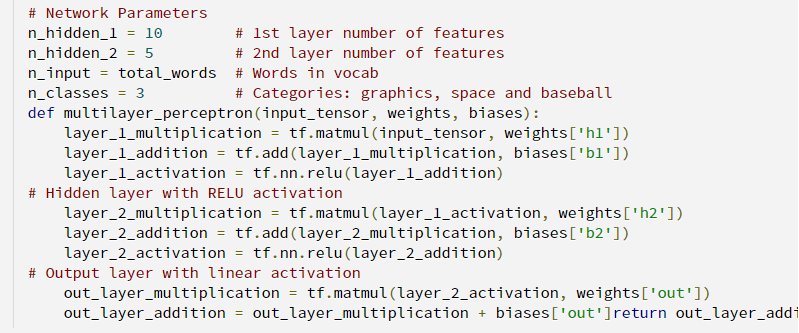Here it calls the matmul() function to realize the multiply function between matrices, and calls the add() function to add the biases into the function.

## 4. How the neural network gets trained:

We can see the main point of the above section is to construct a reasonable structure, and make the weights of the network optimal enough to make the prediction. So the following is to introduce how to train the neural network in TensorFlow. In TensorFlow, we use Variable to store the weights and biases. Here, we should compare the output values with the expected values, and guide the functions to get minimum loss results. There are plenty of methods to calculate the loss function. Since it is a classification task, we should use the cross-entropy error. Prior work by James D. McCaffrey analyzed and concluded the reason to use cross-entropy is to avoid the training stalling out. So we use the cross-entropy error by calling the function: tf.nn.softmax_cross_entropy_with_logits(), we will also calculate the mean error by calling the
function: tf.reduced_mean().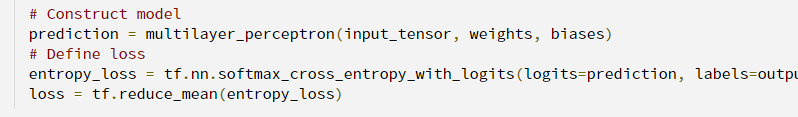We should find the best value to minimize the output error. Here we use stochastic gradient descent (SGD):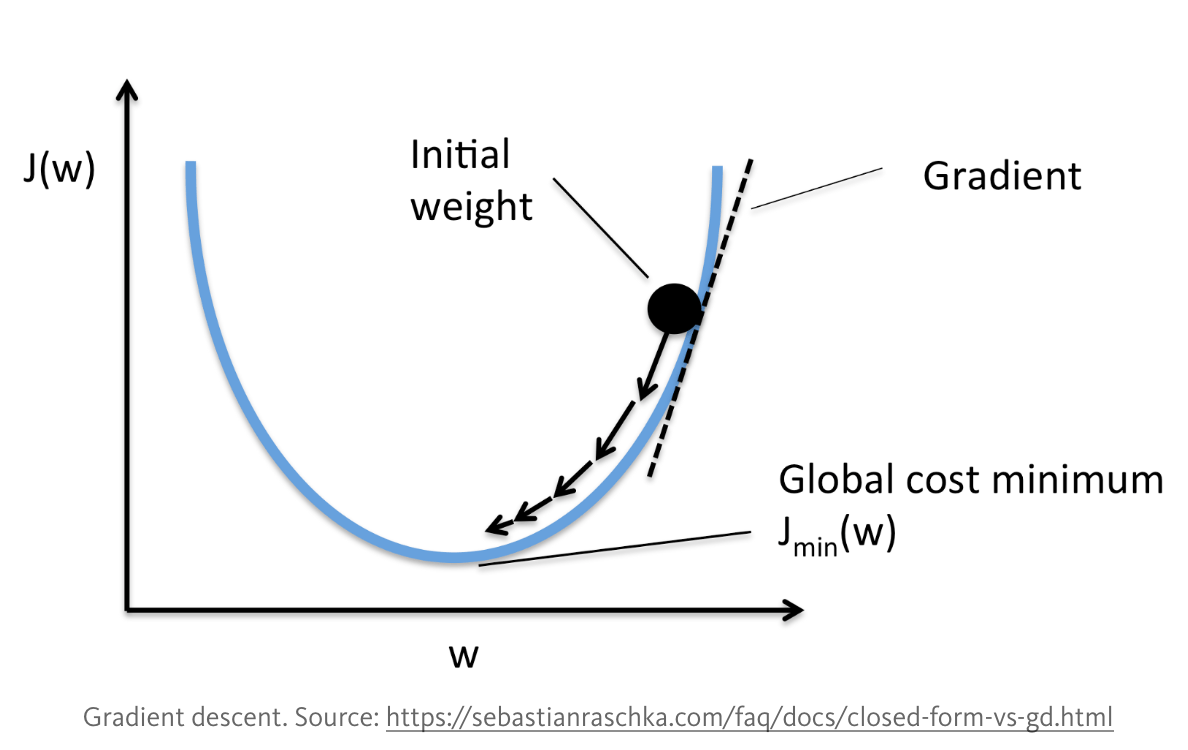Through many iterations, we will get the weights close to the global cost minimum. The learning rate should not be too large. The Adaptive Moment Estimation function is often used to compute the gradient descent. In this optimization algorithm, running averages of both the gradients and the second moments of the gradients are used.

The code is shown as follows, and in other projects, the learning rate can be dynamic which will make the training process faster.## 5. Data manipulation:

This part is also very important for making classification successful. The developers in machine learning should pay more attention to the data. This will save you a lot of time when you want to improve the accuracy of your experiment, because you don’t need to change the configuration from the beginning. Here, the author points out two things that require attention. First, create an index for each word. Then, create a matrix for each text, in which the values are 1 if a word is in the text and 0 otherwise. You can see the code below, as it will help you understand the process.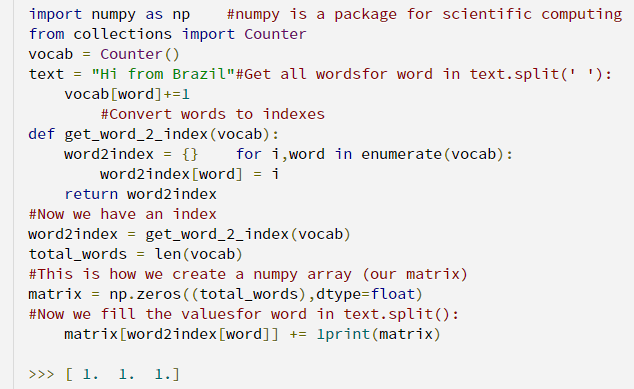Counter() in python is a hash table. When the input is “Hi from Brazil”, the matrix is [1 ,1, 1]. For a different input, which is “Hi”, it will get a different matrix: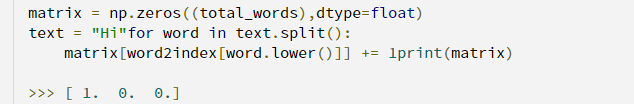## 6. Running and getting the results:

In this part, we will use the 20 Newsgroups as the dataset. It consists of 18,000 posts about 20 topics. The scilit-learn library is used to load this dataset. Here, the author uses 3 categories: comp.graphics, sci.space and rec.sport.baseball. It has two subsets, one for training and one for testing. Below is the way to load the dataset:This follows a pattern, and it’s easy for the developers to use.

In this experiment, the epoch is set at 10, which means there are ten times of forward passes and backward passes to go through the whole dataset. In TensorFlow, the placeholder is defined to serve as the target of feeds, which is used to pass the data for each run step.Here we should separate the training data in batches, because we will feed the dict with a larger batch when testing the model. We call the get_batches() function to get the number of texts with the size of the batch. We can run the model.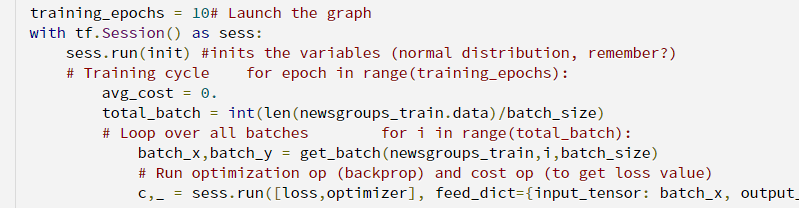Here we should also build the test model and calculate the accuracy.Then we can get the results.## Conclusion:

This article gives us a introduction on how to classify texts with neural networks and TensorFlow. It introduces the basic information that relates to this experiment. The results from running my own version is not as good as the author’s. We can make this architecture deeper, and use the dropout in the hidden layers. This will undoubtedly improve the accuracy.

Also, when you run the code, you should make sure you have the latest version of TensorFlow installed. Sometimes you’ll fail to import the twenty_newsgroups datasets. When this happens, you can use the following code to make it works.The completed code is shown below:

```import pandas as pd
import numpy as np
import tensorflow as tf
from collections import Counter
from sklearn.datasets import fetch_20newsgroups
# if you didn't download the twenty_newsgroups datasets, it will run with error
# this logging can help to solve the error
import logging
logging.basicConfig()

categories = ["comp.graphics","sci.space","rec.sport.baseball"]
newsgroups_train = fetch_20newsgroups(subset='train', categories=categories)
newsgroups_test = fetch_20newsgroups(subset='test', categories=categories)

print('total texts in train:',len(newsgroups_train.data))
print('total texts in test:',len(newsgroups_test.data))

vocab = Counter()
for text in newsgroups_train.data:
for word in text.split(' '):
vocab[word.lower()]+=1

for text in newsgroups_test.data:
for word in text.split(' '):
vocab[word.lower()]+=1

total_words = len(vocab)
def get_word_2_index(vocab):
word2index = {}
for i,word in enumerate(vocab):
word2index[word.lower()] = i

return word2index

word2index = get_word_2_index(vocab)

def get_batch(df,i,batch_size):
batches = []
results = []
texts = df.data[i*batch_size:i*batch_size+batch_size]
categories = df.target[i*batch_size:i*batch_size+batch_size]
for text in texts:
layer = np.zeros(total_words,dtype=float)
for word in text.split(' '):
layer[word2index[word.lower()]] += 1

batches.append(layer)

for category in categories:
y = np.zeros((3),dtype=float)
if category == 0:
y = 1.
elif category == 1:
y = 1.
else:
y = 1.
results.append(y)

return np.array(batches),np.array(results)

# Parameters
learning_rate = 0.01
training_epochs = 10
batch_size = 150
display_step = 1

# Network Parameters
n_hidden_1 = 100 # 1st layer number of features
n_hidden_2 = 100 # 2nd layer number of features
n_input = total_words # Words in vocab
n_classes = 3 # Categories: graphics, sci.space and baseball

input_tensor = tf.placeholder(tf.float32,[None, n_input],name="input")
output_tensor = tf.placeholder(tf.float32,[None, n_classes],name="output")

def multilayer_perceptron(input_tensor, weights, biases):
layer_1_multiplication = tf.matmul(input_tensor, weights['h1'])

# Hidden layer with RELU activation
layer_2_multiplication = tf.matmul(layer_1, weights['h2'])

# Output layer
out_layer_multiplication = tf.matmul(layer_2, weights['out'])

# Store layers weight & bias
weights = {
'h1': tf.Variable(tf.random_normal([n_input, n_hidden_1])),
'h2': tf.Variable(tf.random_normal([n_hidden_1, n_hidden_2])),
'out': tf.Variable(tf.random_normal([n_hidden_2, n_classes]))
}
biases = {
'b1': tf.Variable(tf.random_normal([n_hidden_1])),
'b2': tf.Variable(tf.random_normal([n_hidden_2])),
'out': tf.Variable(tf.random_normal([n_classes]))
}

# Construct model
prediction = multilayer_perceptron(input_tensor, weights, biases)

# Define loss and optimizer
loss = tf.reduce_mean(tf.nn.softmax_cross_entropy_with_logits(logits=prediction, labels=output_tensor))

# Initializing the variables
init = tf.initialize_all_variables()

# Launch the graph
with tf.Session() as sess:
sess.run(init)

# Training cycle
for epoch in range(training_epochs):
avg_cost = 0.
total_batch = int(len(newsgroups_train.data)/batch_size)
# Loop over all batches
for i in range(total_batch):
batch_x,batch_y = get_batch(newsgroups_train,i,batch_size)
# Run optimization op (backprop) and cost op (to get loss value)
c,_ = sess.run([loss,optimizer], feed_dict={input_tensor: batch_x,output_tensor:batch_y})
# Compute average loss
avg_cost += c / total_batch
# Display logs per epoch step
if epoch % display_step == 0:
print("Epoch:", '%04d' % (epoch+1), "loss=", \
"{:.9f}".format(avg_cost))
print("Optimization Finished!")

# Test model
correct_prediction = tf.equal(tf.argmax(prediction, 1), tf.argmax(output_tensor, 1))
# Calculate accuracy
accuracy = tf.reduce_mean(tf.cast(correct_prediction, "float"))
total_test_data = len(newsgroups_test.target)
batch_x_test,batch_y_test = get_batch(newsgroups_test,0,total_test_data)
print("Accuracy:", accuracy.eval({input_tensor: batch_x_test, output_tensor: batch_y_test}))```

## Reference:

Author: Shixin Gu | Localized by Synced Global Team: Junpei Zhong